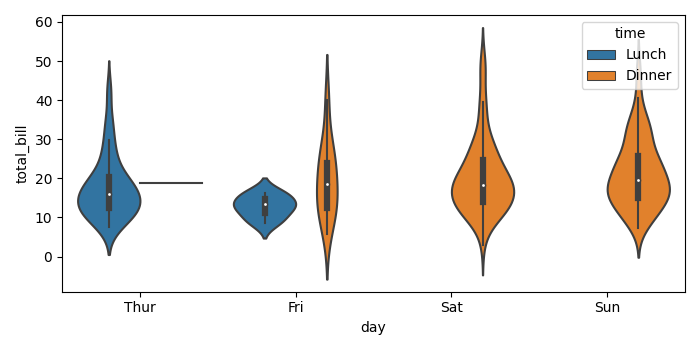# How to plot two violin plot series on the same graph using Seaborn?

To plot two violin plot series on the same graph using Seaborn, we can take the following Steps.

## Steps

• Set the figure size and adjust the padding between and around the subplots.

• Load an example dataset from the online repository (requires Internet).

• Create a violin plot using violinplot() method.

• To display the figure, use Show() method.

## Example

# Import Seaborn and Matplotlib
import seaborn as sns
from matplotlib import pyplot as plt

# Set the figure size
plt.rcParams["figure.figsize"] = [7.00, 3.50]
plt.rcParams["figure.autolayout"] = True

# Create a violin plot using Seaborn
sns.violinplot(x="day", y="total_bill", hue="time", data=tips)

# Display the plot
plt.show()

## Output

It will produce the following output −